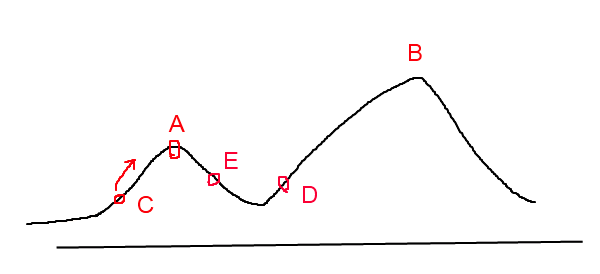## 爬山算法(Hill Climbing)## 模拟退火(SA,Simulated Annealing)思想

模拟退火算法描述：



P(dE)=exp(dE/(kT))

关于爬山算法与模拟退火，有一个有趣的比喻：



## 模拟退火算法伪代码

/*
* J(y)：在状态y时的评价函数值
* Y(i)：表示当前状态
* Y(i+1)：表示新的状态
* r： 用于控制降温的快慢
* T： 系统的温度，系统初始应该要处于一个高温的状态
* T_min ：温度的下限，若温度T达到T_min，则停止搜索
*/
while( T > T_min )
{
dE = J( Y(i+1) ) - J( Y(i) ) ;

if ( dE >=0 ) //表达移动后得到更优解，则总是接受移动
Y(i+1) = Y(i) ; //接受从Y(i)到Y(i+1)的移动
else
{
// 函数exp( dE/T )的取值范围是(0,1) ，dE/T越大，则exp( dE/T )也
if ( exp( dE/T ) > random( 0 , 1 ) )
Y(i+1) = Y(i) ; //接受从Y(i)到Y(i+1)的移动
}
T = r * T ; //降温退火 ，0<r<1 。r越大，降温越慢；r越小，降温越快
/*
* 若r过大，则搜索到全局最优解的可能会较高，但搜索的过程也就较长。若r过小，则搜索的过程会很快，但最终可能会达到一个局部最优值
*/
i ++ ;
}


## 使用模拟退火算法解决旅行商问题

1. 产生一条新的遍历路径P(i+1)，计算路径P(i+1)的长度L(P(i+1))

2. 若L(P(i+1))<L(P(i))，则接受P(i+1)为新的路径，否则以模拟退火的那个概率接受P(i+1)，然后降温

3. 重复步骤1，2直到满足退出条件


1. 随机选择2个节点，交换路径中的这2个节点的顺序。

2. 随机选择2个节点，将路径中这2个节点间的节点顺序逆转。

3. 随机选择3个节点m，n，k，然后将节点m与n间的节点移位到节点k后面。


package com.test1;

import java.lang.reflect.Array;
import java.util.*;

public class SimulatedAnnealing {

public static void main(String[] args) {
SA sa=new SA();
int[][] test=sa.getData();
int[][] test2=sa.getMatrix(6);
sa.sa(test2, 2);
}
}
class SA{
//随机获取一个矩阵数据表示个城市之间的距离
public int[][] getMatrix(int cityNum){
Random random=new Random();
int[][] matrix=new int[cityNum][cityNum];
for(int i=0;i<cityNum;i++){
matrix[i][i]=999;
}
for(int i=0;i<cityNum;i++){
for(int j=i+1;j<cityNum;j++){
matrix[i][j]=random.nextInt(100);
matrix[j][i]=matrix[i][j];
}
}
return matrix;
}
//随时选择两个城市交换位置，生成新的路径
public List getNewPath(List path){
Random random=new Random();
int size=path.size();
if(size<2){
System.out.println("城市数目太少，无法生成新的路径");
return path;
}
int position1;
int position2;
do{
position1=random.nextInt(size-1);
position2=random.nextInt(size-1);
}while(position1==0 || position2==0 || position1==position2);

Integer temp=(Integer)path.get(position1);he xo
path.set(position1,(Integer)path.get(position2));
path.set(position2,temp);

return path;
}
//计算接受的概率
public double acceptanceProbability(int length,int newLength,double temperature){
if(newLength < length){
return 1.1;
}
return Math.exp((length-newLength)/temperature);
}
//模拟退火过程，得到最优方案
public List sa(int[][] matrix,int start){
//record the number of cycles
int num=1;
//初始化温度
double temperature=1000;
//冷却概率
double coolingRate=0.997;
//初始化解决方案
//List firstPath=greedy(matrix,1);
List firstPath=getFirstPath(matrix.length,start);
List bestPath=firstPath;
System.out.print("第0次： path:");
printPath(bestPath);
System.out.print(" length:"+getLength(matrix, bestPath)+"\n");
//循环直至系统冷却
while(temperature>1){
//get current path's length
int length=getLength(matrix, bestPath);
//create a new path
List newPath=getNewPath(bestPath);
//get newPath's length
int newLength=getLength(matrix, newPath);
//decition
double acceptanceProbability=acceptanceProbability(length, newLength, temperature);
if(acceptanceProbability>Math.random()){
//acceptance
bestPath=newPath;
System.out.print("第"+num+"次： path:");
printPath(bestPath);
System.out.print(" length:"+getLength(matrix, bestPath)+"\n");
num++;
}else{
//give up the new path
}
//cooling
temperature*=coolingRate;
}
return bestPath;
}
//使用贪心算法获得一个初始的遍历序列
public List greedy(int[][] matrix,int start){
int line=matrix.length;
if(start>(line-1)){
System.out.println("输入数据有误！");
return null;
}
int point_now=start;
for(int i=0;i<(line-1);i++){
int min=999;
int next_point=0;
for(int j=0;j<line;j++){
min=matrix[point_now][j];
next_point=j;
}
}
point_now=next_point;
}
}
//init the first path
public List getFirstPath(int cityNum,int start){
List path=new ArrayList();
for(int i=1;i<cityNum;i++){
if(i!=start)
}
return path;
}
//计算一个路径的总长度
public int getLength(int[][] matrix,List path){
int path_length=0;
for(int i=0;i<path.size()-1;i++){
path_length+=matrix[(Integer)path.get(i+1)][(Integer)path.get(i)];
}
return path_length;
}
//得到一个测试数据
public static int[][] getData(){
int[][] data=new int;
data=new int[]{999,48,49,90,42};
data=new int[]{48,999,24,22,49};
data=new int[]{49,24,999,65,65};
data=new int[]{90,22,65,999,92};
data=new int[]{42,49,65,92,999};
return data;
}
//print a path
public void printPath(List path){
for(int i=0;i<path.size();i++){
System.out.print(path.get(i)+" ");
}
}
}